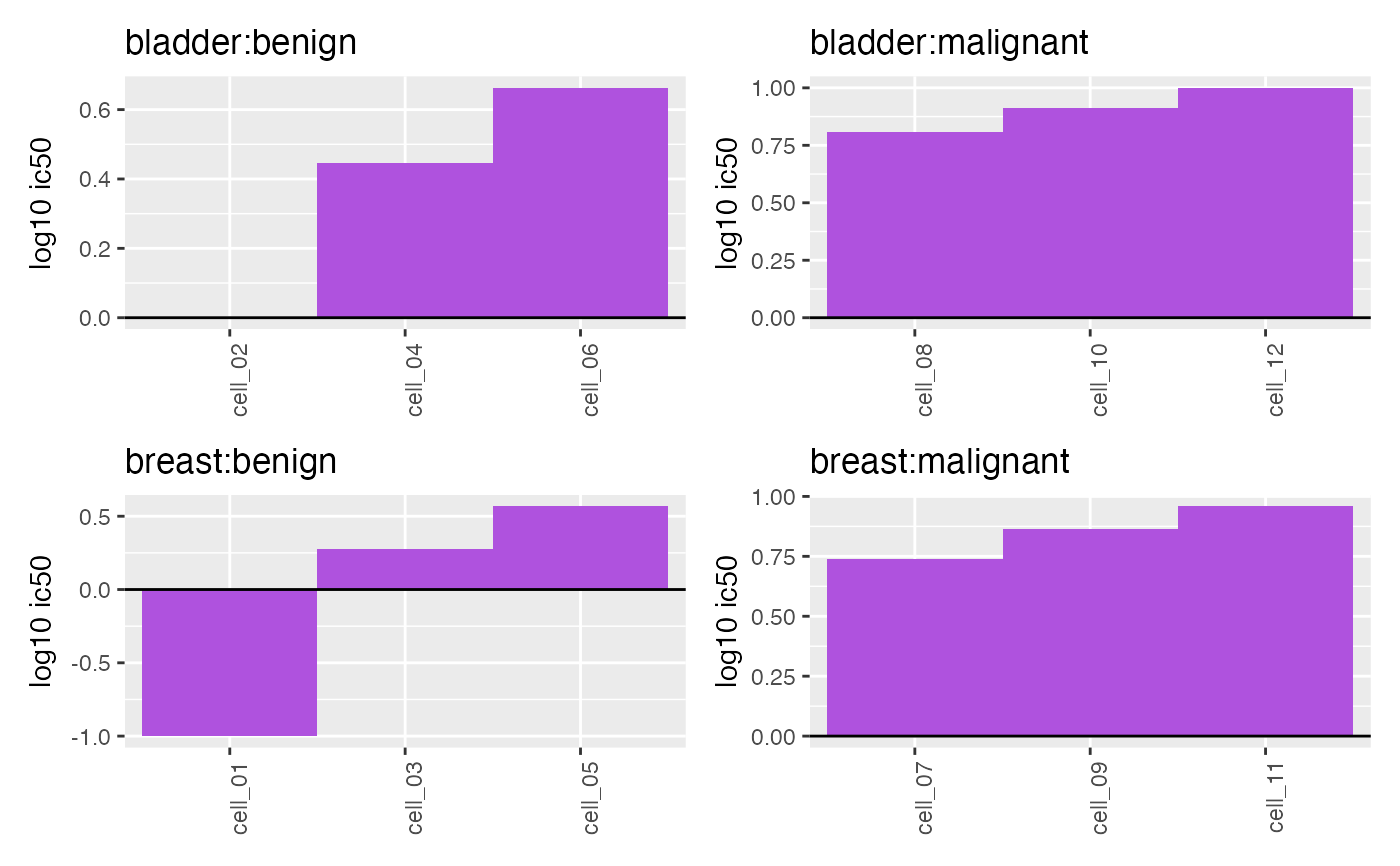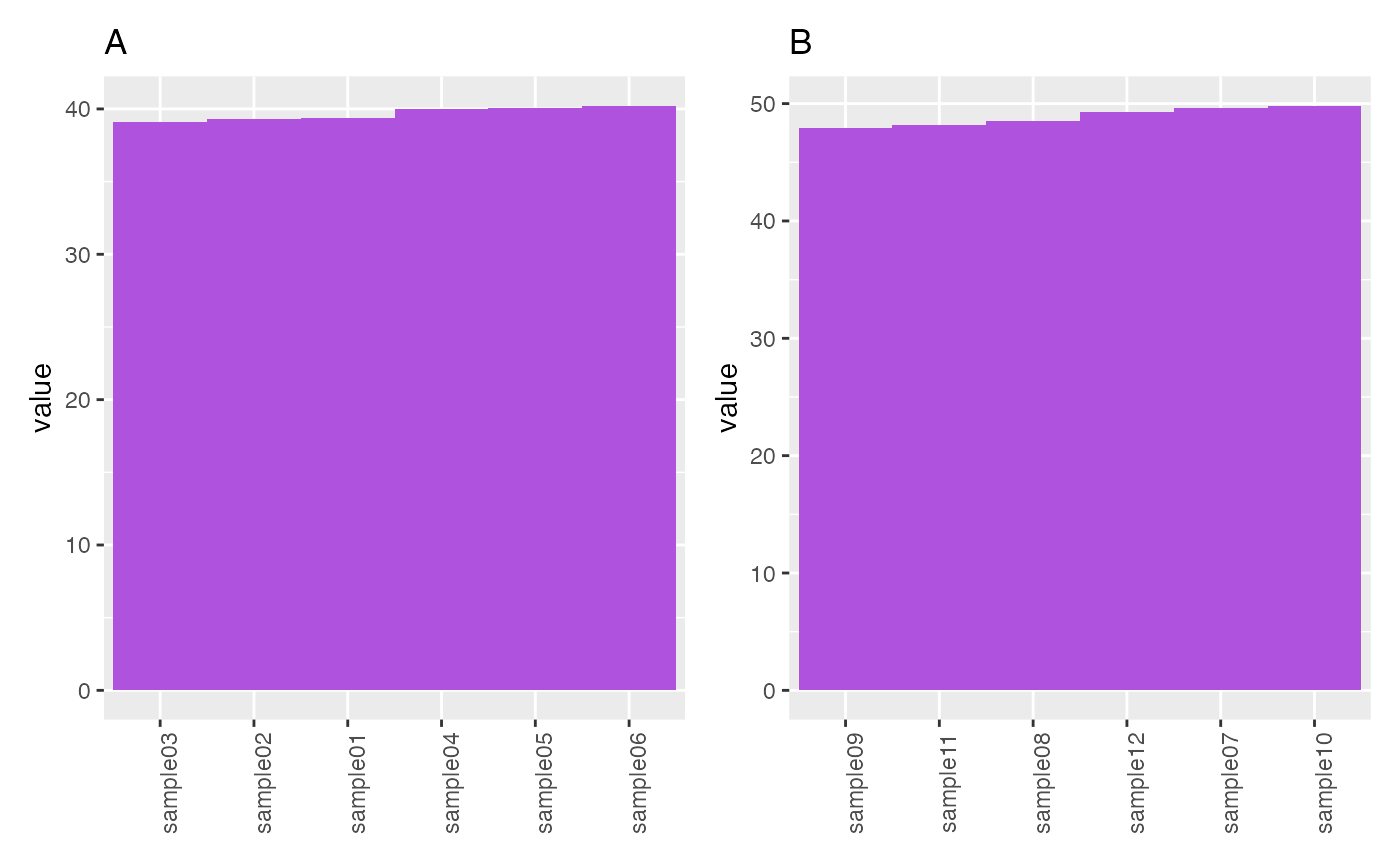Plot waterfall

## Usage

plotWaterfall(object, ...)

# S4 method for DFrame
plotWaterfall(
object,
sampleCol,
valueCol,
interestingGroups = NULL,
trans = c("identity", "log2", "log10"),
fill = purpleOrange(1L),
labels = list(title = NULL, subtitle = NULL)
)

# S4 method for SummarizedExperiment
plotWaterfall(
object,
assay = 1L,
fun = c("mean", "sum"),
interestingGroups = NULL,
...
)

# S4 method for data.frame
plotWaterfall(
object,
sampleCol,
valueCol,
interestingGroups = NULL,
trans = c("identity", "log2", "log10"),
fill = purpleOrange(1L),
labels = list(title = NULL, subtitle = NULL)
)

## Arguments

object

Object.

sampleCol

character(1). Column name of discrete samples to plot on X axis.

valueCol

character(1). Column name of continues values to plot on Y axis.

interestingGroups

character. Groups of interest to use for visualization. Corresponds to factors describing the columns of the object.

trans

character(1). Name of the axis scale transformation to apply.

help(topic = "scale_x_continuous", package = "ggplot2")

fill

character(1). R color name or hex color code (e.g. "#AF52DE").

labels

list. ggplot2 labels. See ggplot2::labs() for details.

assay

vector(1). Assay name or index position.

fun

function.

...

## Value

ggplot.

## Note

Updated 2023-08-10.

## Examples

data(RangedSummarizedExperiment, package = "AcidTest")

## data.frame ====
object <- data.frame(
object = paste("cell", seq_len(12L), sep = "_")
),
"ic50" = seq(
from = 0.1,
to = 10L,
length.out = 12L
),
"tumorType" = rep(
times = 6L
),
"tumorSubtype" = rep(
x = c("benign", "malignant"),
each = 6L
)
)
plotWaterfall(
object = object,
sampleCol = "cellId",
valueCol = "ic50",
interestingGroups = c("tumorType", "tumorSubtype"),
trans = "log10"
)## SummarizedExperiment ====
object <- RangedSummarizedExperiment
plotWaterfall(object, trans = "identity")